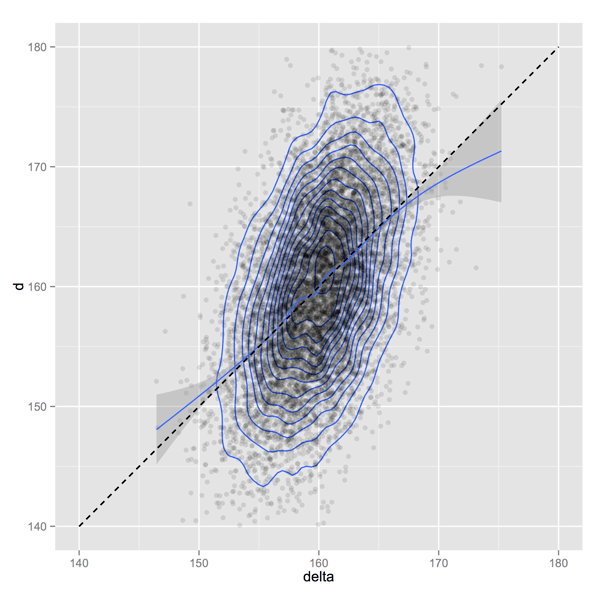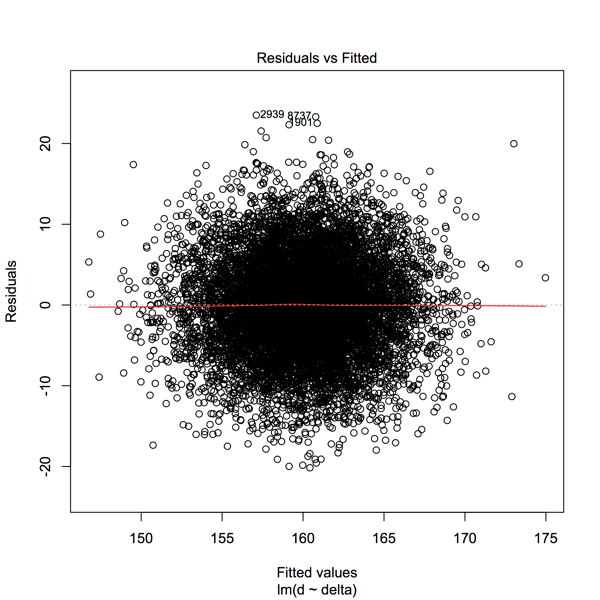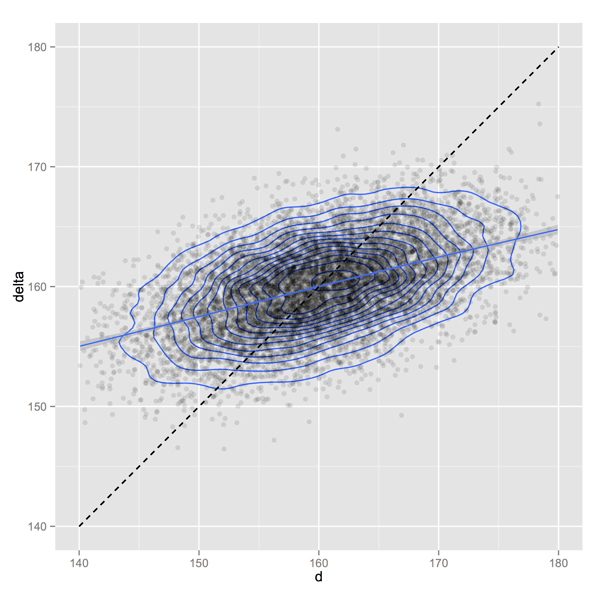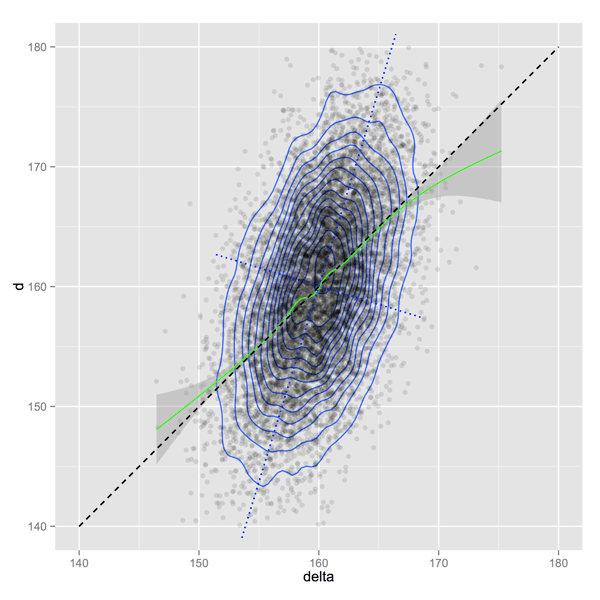# Automatic bias correction doesn’t fix omitted variable bias

Page 94 of Gelman, Carlin, Stern, Dunson, Vehtari, Rubin “Bayesian Data Analysis” 3rd Edition (which we will call BDA3) provides a great example of what happens when common broad frequentist bias criticisms are over-applied to predictions from ordinary linear regression: the predictions appear to fall apart. BDA3 goes on to exhibit what might be considered the kind of automatic/mechanical fix responding to such criticisms would entail (producing a bias corrected predictor), and rightly shows these adjusted predictions are far worse than the original ordinary linear regression predictions. BDA3 makes a number of interesting points and is worth studying closely. We work their example in a bit more detail for emphasis.

An easy way to avoid fairly evaluating an analysis technique is to assert that the technique in question is unsound because it violates some important foundational axiom of sound analysis. This rapidly moves a discussion from a potentially difficult analysis to an easy debate. However, this (unfortunately common) behavior is mere gamesmanship (see Potter “The Theory and Practice of Gamesmanship (or the Art of Winning Games without Actually Cheating)”). But it is what you can encounter when presenting a technique from school “B” to members of school “A.” For example: Bayesian parameter estimates can be considered inadmissible by frequentists because the estimates may be biased (see Frequentist inference only seems easy for an interesting example of the principle, and of a valid low-variance estimate that is in necessarily biased). BDA3 page 94 provides an interesting situation with a deliberate omitted variable bias (a feature of the data). BDA3 goes on to demonstrates how silly it would be to apportion the blame for prediction bias to the inference technique used (ordinary linear regression), or to try and mechanically adjust for prediction bias it without fixing the underlying omitted variable issue (by recruiting more variables/features). The example is important because, as we demonstrated in our earlier article: so-called unbiased techniques work by rejecting many (possibly good) biased estimates, and therefore can implicitly incorporate potentially domain-inappropriate bias corrections or adjustments. This example is relevant, because it is easier to respond to such criticism when it applied to a standard technique used on a simple artificial problem (versus defending a specialized technique on messy real data).

Axiomatic approaches to statistical inference tend to be very brittle in that it takes only a few simple rules to build an paradoxical or unsatisfiable system. For example: we described how even insisting on the single reasonable axiom of unbiasedness completely determines a family of statistical estimates (leaving absolutely no room to attempt to satisfy any additional independent conditions or axioms).

This sort of axiomatic brittleness is not unique to statistical inference. It is a common experience that small families of seemingly reasonable (and important) desiderata lead to inconsistent and unsatisfiable systems when converted to axioms. Examples include Arrow’s impossibility theorem (showing a certain reasonable combination of goals in voting systems is unachievable), Brewer’s CAP theorem (showing a certain reasonable combination of distributed computing goals are mutually incompatible). So the reason a given analysis may not satisfy an obvious set of desirable axioms is often that no analysis satisfies the given set of axioms.

Let’s get back to the BDA3 example and work out how to criticize ordinary linear regression for having an undesirable bias. If linear regression can’t stand up to this sort of criticism, how can any other method to be expected to face the same? If we are merely looking at the words it is “obvious” that regression can’t be biased as this would contradict the Gauss-Markov theorem (that linear regression is the “best linear unbiased estimator” or BLUE). However, the word “bias” can have different meanings in different contexts: in particular what is biased with respect to what? Let’s refine the idea of bias and try to make ordinary linear regression look bad.

Consider the following simple problem. Suppose our data set is observations of pairs of mother’s and adult daughter’s heights. Suppose idealizations of these two random variables are generated by the following process:

1. `c` (unobserved) is independently sampled from a normal distribution with a mean of 80 centimeters and a standard deviation of 5 centimeters (the shared or common component of height).
2. `u` (unobserved) is independently sampled from a normal distribution with a mean of 80 centimeters and a standard deviation of 5 centimeters (the unique to mother portion of height).
3. `v` (unobserved) is independently sampled from a normal distribution with a mean of 80 centimeters and a standard deviation of 5 centimeters (the unique to daughter portion of height).
4. We then observe the two derived random variables: mother’s height `m=c+u`, and adult daughter’s height `d=c+v`.

The random variables `m` and `d` are normally distributed with equal means of 160 centimeters, equal variances, and a correlation of 0.5. As we said: we can think of the two random variables `m` and `d` as representing the heights of pairs of mothers and adult daughters. The correlation means tall mothers tend to have taller daughters (but the correlation being less that 1.0 means the mother’s height does not completely determine the daughter’s height). Obviously real heights are not normally distributed (as people do not have negative heights, and non-degenerate normal distributions have non-zero mass on negative values); but overall the normal distribution is a very good approximation of plausible heights.

This generative model represents a specialization of the example from BDA3 page 94 to specific distributions that clearly obey the properties claimed in BDA3. We are completely specifying the distributions to attempt to negate any (wrong) claim that there may not be distributions simultaneously having all of the claimed properties mentioned in the original BDA3 example. The interpretation (again from BDA3) of the two observed random variables `m` and `d` as pairs of mother/daughter heights is to give the data an obvious interpretation and help make obvious when our procedures become silly. At this point we have distributions exactly matching the claimed properties in BDA3 and very closely (but not exactly) matching the claimed interpretation as heights of pairs of mothers and their adult daughters.

Let’s move on to the analysis. The claim in BDA3 is that the posterior mean of `d` given `m` is:
`E[d|m] = 160 + 0.5 (m-160)`. We could derive this through Bayes law and some calculus/algebra. But we get the exact same answer using ordinary linear regression (which tends to have a frequentist justification). In R:

``` n <- 10000 set.seed(4369306) d <- data.frame(c=rnorm(n,mean=80,sd=5), u=rnorm(n,mean=80,sd=5), v=rnorm(n,mean=80,sd=5)) d\$m <- d\$c+d\$u d\$d <- d\$c+d\$v print(cor(d\$m,d\$d)) ##  0.4958206 print(lm(d~m,data=d)) ## ## Call: ## lm(formula = d ~ m, data = d) ## ## Coefficients: ## (Intercept) m ## 81.6638 0.4899 ```

The recovered linear model is very close to the claimed theoretical conditioned expectation `E[d|m] = 160 + 0.5 (m-160) = 80 + 0.5 m`. So we can assume a good estimate of `d` can be learned from the data. To keep things neat let’s say our point-estimate for `d` is called `δ`, and `δ = 160 + 0.5 (m-160)`. As we see below `δ` is a plausible looking estimate:

``` library(ggplot2) d\$delta <- 160 + 0.5*(d\$m-160) ggplot(data=d,aes(x=delta,y=d)) + geom_density2d() + geom_point(alpha=0.1) + geom_smooth() + geom_segment(x=150,xend=170,y=150,yend=170,linetype=2) + coord_equal(ratio=1) + xlim(140,180) + ylim(140,180) ```Actuals (`d`) plotted against estimate `δ`

Notice the dashed line `y=x` mostly coincides with the blue smoothing curve in the above graph; this is a visual confirmation that `E[d|δ] = δ`. This follows because we chose `δ` so that `δ = E[d|m]` (i.e. matching the regression estimate) and if we know `δ` then we (by simple linear substitution) also know `m`. So `E[d|δ] = E[d|m] = δ`. `E[d|δ] = δ` seems like a very nice property to have for the estimate `δ` to have. We can (partially) re-confirm it by fitting a linear model of `d` as a linear function of `δ`:

``` print(summary(lm(d~delta,data=d))) ## Call: ## lm(formula = d ~ delta, data = d) ## ## Residuals: ## Min 1Q Median 3Q Max ## -20.1532 -4.1458 0.0286 4.1461 23.5144 ## ## Coefficients: ## Estimate Std. Error t value Pr(>|t|) ## (Intercept) 3.28598 2.74655 1.196 0.232 ## delta 0.97972 0.01716 57.089 <2e-16 *** ## --- ## Signif. codes: 0 ‘***’ 0.001 ‘**’ 0.01 ‘*’ 0.05 ‘.’ 0.1 ‘ ’ 1 ## ## Residual standard error: 6.126 on 9998 degrees of freedom ## Multiple R-squared: 0.2458, Adjusted R-squared: 0.2458 ## F-statistic: 3259 on 1 and 9998 DF, p-value: < 2.2e-16 ```

We see the expected slope near one and an intercept/dc-term statistically indistinguishable from zero. And we don’t really have much bad to say about this fit (beyond the R-squared of 0.2458, which is expected when correlation is known to be 0.5). For instance the residuals don’t formally appear structured (despite the obvious visible tilt of principal axes in the previous graph):

``` plot(lm(d~delta,data=d)) ```And now for the (intentionally overreaching) frequentist criticism. From BDA3 page 94 (variable names changed): “The posterior mean is not, however, an unbiased estimate of `d` in the sense of repeated sampling of `m` for a fixed `d`.” That is: the chosen estimate `δ` is not an unbiased estimate of a general fixed unknown value of `d` under repeated experiments where the observed variable `m` varies according to repeated draws from the joint distribution. This may sound complicated, but it is the standard frequentist definition of an unbiased estimator: for any given fixed unknown value of the item to be estimated under repeated experiments (with new, possibly different observed data) the value of the estimate should match the fixed unknown value in expectation. In other words: it isn’t considered enough for a single given estimate `δ` to capture the expected value of the unknown item `d` (to have `E[d|δ] = δ`, which we have confirmed), we must also have the whole estimation procedure be unbiased for arbitrary unknown `d` (that would be `E[δ|d] = d`, which we will show does not hold in general). To be clear BDA3 is not advocating this criticism, they are just citing it as a standard frequentist criterion often wrongly over-applied to methods designed with different objectives in mind. The punch-line is: the predictions from the method of ordinary linear regression fail this criticism, yet the method continues to stand.

Let’s confirm `E[δ|d] ≠ d` in general. To do this we need one more lemma: for a fixed (unknown) value of `d` we know the conditional expectation of the observable value of `m` is `E[m|d] = 160 + 0.5 (d-160)`. We can again get this by a Bayesian argument, or just by running the linear regression `lm(m~d,data=d)` and remembering that linear regression is a linear estimate of the conditional expectation. We are now ready to look at the expected value of our estimate `δ` conditioned on the unknown true value `d`: `E[δ|d]`. Plugging in what we know we get:

``` E[δ|d] = E[160 + 0.5 (m-160) | d] = 160 + 0.5 (E[m|d]-160) = 160 + 0.5 ((160 + 0.5 (d-160))-160) = 160 + 0.25 (d - 160) ```

And that is a problem. To satisfy frequentist unbiasedness we would need `E[δ|d] = d` for all `d`. And `160 + 0.25 (d - 160) = d` only if `d=160`. So for all but one possible value of the daughter’s height `d` the ordinary linear regression’s prediction procedure is considered biased in the frequentist sense. In fact we didn’t actually use the regression coefficients, we used the exact coefficients implied by the generative model that is actually building the examples. So we could even say: using the actual generative model to produce predictions is not unbiased in the frequentist sense.

This would seem to contradict our earlier regression check, but that is not the case. Consider the following regression and graph:

``` ```
``````print(summary(lm(delta~d,data=d)))

## Call:
## lm(formula = delta ~ d, data = d)
##
## Residuals:
##      Min       1Q   Median       3Q      Max
## -12.4663  -2.0743  -0.0216   2.0890  12.7414
##
## Coefficients:
##              Estimate Std. Error t value Pr(>|t|)
## (Intercept) 1.198e+02  7.041e-01  170.20   <2e-16 ***
## d           2.509e-01  4.395e-03   57.09   <2e-16 ***
## ---
## Signif. codes:  0 ‘***’ 0.001 ‘**’ 0.01 ‘*’ 0.05 ‘.’ 0.1 ‘ ’ 1
##
## Residual standard error: 3.1 on 9998 degrees of freedom
## Multiple R-squared:  0.2458,	Adjusted R-squared:  0.2458
## F-statistic:  3259 on 1 and 9998 DF,  p-value: < 2.2e-16

ggplot(data=d,aes(x=d,y=delta)) +
geom_density2d() + geom_point(alpha=0.1) +
geom_smooth() +
geom_segment(x=140,xend=180,y=140,yend=180,linetype=2) +
coord_equal(ratio=1) + xlim(140,180) + ylim(140,180)
```
```Estimate `δ` plotted against actuals (`d`)

Notice that the slope (both from the regression and the graph) is now 0.25 and the dashed "y=x" line no longer agrees with the empirical smoothing curve. This closely agrees with our derived form for `E[δ|d]`. And this may expose one source of the confusion. The slope of the regression `d ~ δ` is 1.0, while the slope of the regression `δ ~ d` is 0.25. This violates a possible naive expectation/intuition that these two slopes should be reciprocal (which they need not be, as each has a different error model).

Part of what is going on is an expected reversion to the mean effect. If we have a given `m` in hand then `δ = 160 + 0.5 (m-160)` is in fact a good estimate for `d` (given that we known only `m` and don't have a direct better estimate for `c`). What we don't have is a the ability to guess what part of the heights to be estimated is from the shared process (`c`, which we can consider an omitted variable in this simple analysis) and what part is from the unique processes (`u` and `v`, and therefore not useful for prediction).

One concern: we have been taking all conditional expectations `E[|]` over the same data set (a nice single consistent probability model). This doesn't quite reproduce the frequentist set-up of `d` being fixed. However, if there was in fact no reversion to the mean on any `d`-slice then we would not have seen reversion to the mean in the aggregate. We can check the fixed-`d` case directly with a little math to produce new fixed-`d` data set, or approximate it by censoring a larger data set down to a narrow interval of `d`. Here is a such an example (showing the same effects we saw before):

``` n2 <- 10000000 set.seed(4369306) d2 <- data.frame(c=rnorm(n2,mean=80,sd=5), u=rnorm(n2,mean=80,sd=5), v=rnorm(n2,mean=80,sd=5)) d2\$m <- d2\$c+d2\$u d2\$d <- d2\$c+d2\$v d2 <- subset(d2,d>=170.1 & d<170.2) d2\$delta <- 160 + 0.5*(d2\$m-160) print(dim(d2)[]) ##  20042 print(mean(d2\$d)) ##  170.1498 print(mean(d2\$delta)) ##  162.5718 print(mean(160 + 0.25*(d2\$d-160))) ##  162.5374 ```

And we pretty much see the exact reversion to the mean expected from our derivation.

Back to the Gauss-Markov theorem: in what sense can ordinary linear regression be considered unbiased? It turns out if you read carefully the content of the Gauss-Markov theorem is that the estimates of unknown parameters (or betas) are unbiased. So in particular the estimate `lm(d~m,data=d)` should recover coefficients that are unbiased estimates of the coefficients in the expression `E[d|m] = 160 + 0.5 (m-160)`. And that appears plausible, as we empirically estimated `d ~ 81.6638 + 0.4899*m` which is very close to the true values. The Gauss-Markov theorem says ordinary linear regression, given appropriate assumptions, gives us unbiased estimates of models. It does not say that evaluations of such model are themselves unbiased (in the frequentist sense) predictions of instances. In fact, as we have seen, even evaluations of the exact true model does not always give unbiased (in the frequentist sense) predictions for individual instances. This is one reason that frequentist analysis has to take some care in treating unobservable parameters and unobserved future instances very differently (supporting the distinction between prediction and estimation, less of a concern in Bayesian analysis). This also is a good reminder of the fact that traditional statistics is much more interested in parameter estimation, than in prediction of individual instances.

BDA3 goes on to exhibit (for the purpose of criticism) the mechanical derivation of a frequentist-sense unbiased linear estimator for `d`: `γ = 160 + 2 (m-160)`. It is true that `γ` satisfies the unbiased condition `E[γ|d] = d` for all `d`. But `γ` is clearly an unusable and ridiculous estimator that claims for every centimeter in height increase in the `m` mother we should expect two centimeters of expected height increase in the daughter. This is not an effect seen in the data (so not something a good estimator should claim) and is a much higher variance estimator than the common reasonable estimator `δ`. A point BDA3 is making is: applying "bias corrections" willy-nilly or restricting to only unbiased predictors is an ill-advised attempt at a mechanical fix to modeling bias. When the underlying issue is omitted variable bias (as it is in this example) the correct fix is to try and get better estimates of the hidden variables (in this case `c`) by introducing more explanatory variables (in this case perhaps obtaining some genetic and diet measurements for each mother/daughter pair).

So: a deliberately far too broad application of a too stringent frequentist bias condition eliminated reasonable predictors leaving us with a bad one. The fact is: unbiasedness is a stronger condition than is commonly thought, and can limit your set of possible solutions very dramatically (as was also shown in our earlier example). Bias is bad (it can prevent you from improving results through aggregation), but you can't rely on mere mechanical procedures to eliminate it. Correctly controlling for bias may involve making additional measurments and introducing new variables. You also really need to be allowed to examine the domain specific utility of your procedures, and not be told a large number of them are a-priori inadmissible.

We took the term inadmissible from discussions about the James-Stein estimator. One of those results shows: "The ordinary decision rule for estimating the mean of a multivariate Gaussian distribution is inadmissible under mean squared error risk" (from Wikipedia: Stein's example). Though really what this shows is that insisting only on "admissible estimators" (a loaded term if there ever was one) collapses under its own weight (linear regression in many cases actually being a good method for prediction). So such criticism of criticisms are already well known, but evidently not always sufficiently ready to hand.

Afterthought: the plots we made looked a lot like the cover of Freedman, Pisani, Purves "Statistics" 4th edition (which itself is likely a reminder that the line from regressing `y~x` is not the same as the principal axes). So in this example we see the regression line `y~x` is not necessarily the transpose or reciprocal of the regression line `x~y`, and neither of these is necessarily one of the principal axes of the scatterplot.

To follow up on this we produced some plots showing regression lines, smoothed curves, y=x, and principal axes all at once. The graphs are bit too busy/confusing for the main part of of the article itself, but nice to know how to produce (for use debugging, and during data exploration). We have also changed the smoothing curve to green, to give it a chance to stand out from the other annotations.

``` # build some ggplot2() line segments representing principal axes pcompAxes <- function(x,y) { axes <- list() means <- c(mean(x),mean(y)) dp <- data.frame(x=x-means,y=y-means) p <- prcomp(~x+y,data=dp) for(j in 1:2) { s <- p\$rotation[,j] step <- 3*p\$sdev[j] a = means - step*s b = means + step*s axes[[length(axes)+1]] <- geom_segment(x=a,xend=b,y=a,yend=b, color='blue',linetype=3) } axes } ggplot(data=d,aes(x=delta,y=d)) + geom_density2d() + geom_point(alpha=0.1) + geom_smooth(color='green') + geom_segment(x=140,xend=180,y=140,yend=180,linetype=2) + coord_equal(ratio=1) + xlim(140,180) + ylim(140,180) + pcompAxes(d\$delta,d\$d) `````` ggplot(data=d,aes(x=d,y=delta)) + geom_density2d() + geom_point(alpha=0.1) + geom_smooth(color='green') + geom_segment(x=140,xend=180,y=140,yend=180,linetype=2) + coord_equal(ratio=1) + xlim(140,180) + ylim(140,180) + pcompAxes(d\$d,d\$delta) ```### jmount

Data Scientist and trainer at Win Vector LLC. One of the authors of Practical Data Science with R.

1.jmount says: